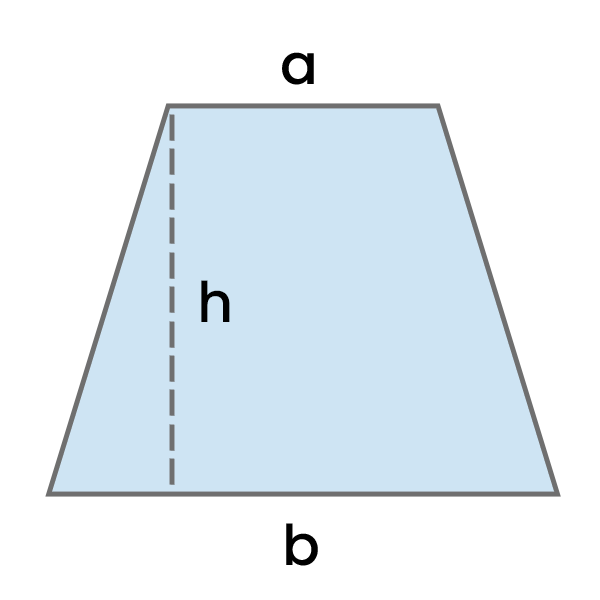# Area of a Trapezoid Calculator

Calculate the area of a trapezoid with given lengths of sides and height in selected units.Result:

## How to use this calculator?

1. Enter the Side length (a) in the selected unit.
2. Enter the Side length (b) in the selected unit.
3. Enter the Height (h) in the selected unit.
4. See the calculated Area of a Trapezoid in the Result section.

## How to calculate Area of a Trapezoid?

Add the lengths of sides a and b, and then divide the result by 2. Take this number and multiply it by the height of the trapezoid (h).

Area of a Trapezoid = (a + b) / 2 * h

Where:

• a - Side Length (a)
• b - Side Length (b)
• h - Height (h)
Example: Calculate the area of a trapezoid with side length (a) of 7 cm, side length (b) of 10 cm, and height of 8 cm.

Area = (a + b) / 2 * h

Area = (7 cm + 10 cm) / 2 * 8 cm

Area = 17 cm / 2 * 8 cm

Area = 8.5 cm * 8 cm

Area = 8.5 cm * 8 cm = 68 cm²

Math Calculators
PureTables.com
Have an idea for a tool or calculator that would make your life easier? Contact us, so we could try to create and provide it.